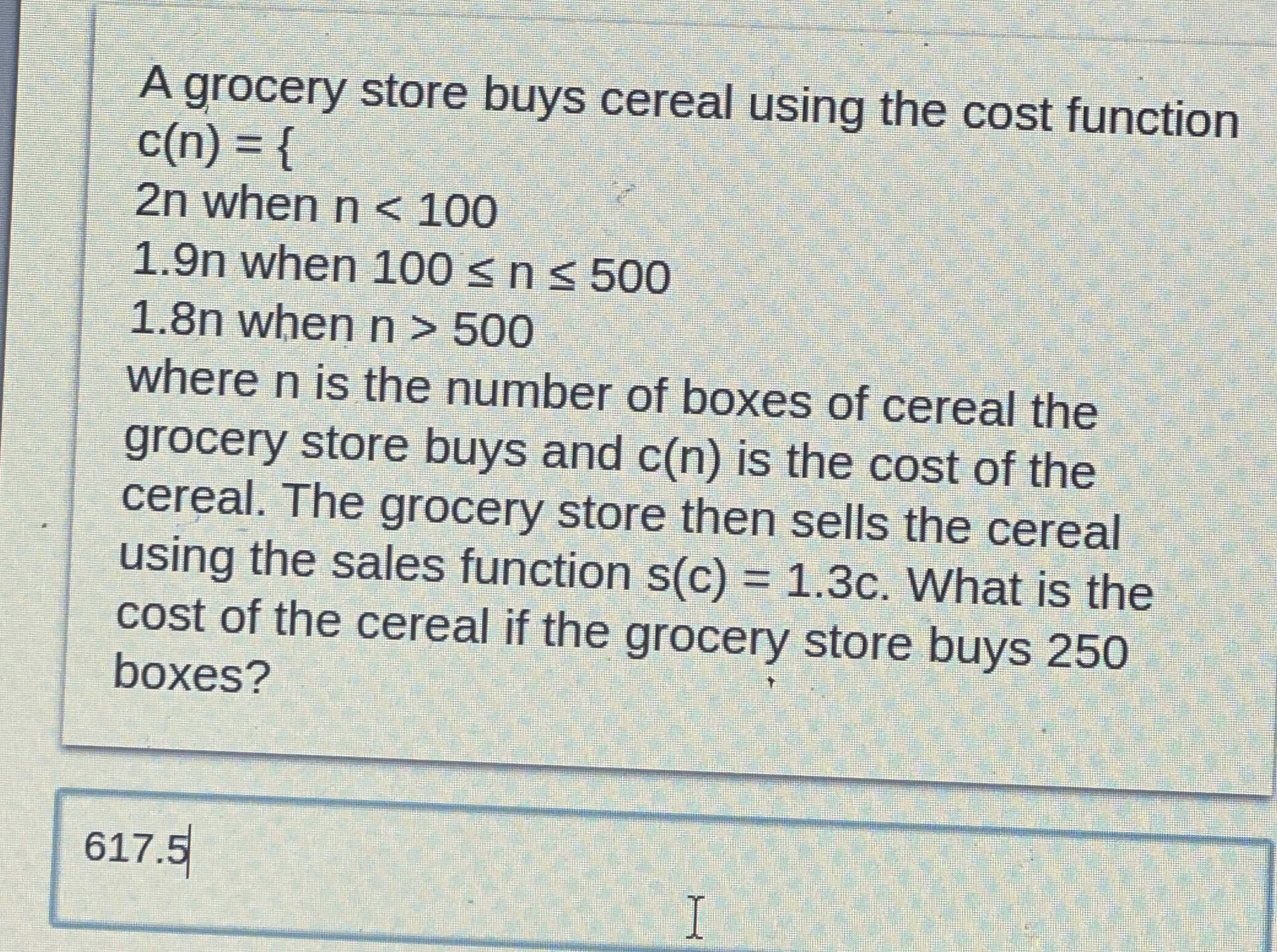### ¿Todavía tienes preguntas de matemáticas?

Pregunte a nuestros tutores expertos
Algebra
PreguntaA grocery store buys cereal using the cost function

$$c ( n ) = \{$$

$$2 n$$ when $$n < 100$$

$$1.9 n$$ when $$100 \leq n \leq 500$$

$$1.8 n$$ when $$n > 500$$

where $$n$$ is the number of boxes of cereal the grocery store buys and $$c ( n )$$ is the cost of the cereal. The grocery store then sells the cereal using the sales function $$s ( c ) = 1.3 c$$ . What is the cost of the cereal if the grocery store buys $$250$$ boxes?

$$617.5$$